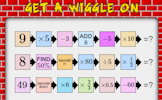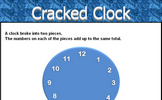# Multiplicative change

### Term 1 starting in week 3 :: Estimated time: 2 weeks

• Solve problems involving direct proportion
• Explore conversion graphs
• Convert between currencies
• Explore direct proportion graphs
• Explore relationships between similar shapes
• Understand scale factors as multiplicative representations
• Draw and interpret scale diagrams
• Interpret maps using scale factors and ratios

This page should remember your ticks from one visit to the next for a period of time. It does this by using Local Storage so the information is saved only on the computer you are working on right now.

## Lesson Starters

Here are some suggestions for whole-class, projectable resources which can be used at the beginnings of each lesson in this block.

### 1st Lesson#### Consecutive Squares

What do you notice about the difference between the squares of consecutive numbers?

### 2nd Lesson#### ClockEquate

Can you use the digits on the left of this clock along with any mathematical operations to equal the digits on the right?

### 3rd Lesson#### Get A Wiggle On

A mixture of calculations to get your brain working at the start of a mathematics lesson.

### 4th Lesson#### Cracked Clock

How did the clock break if the numbers on each of the pieces added up to the same total?

### 5th Lesson#### Cross Perimeter

Calculate the distance around the given shape

### 6th Lesson#### Rows of Roses

Can you draw 4 straight lines, without taking your pencil off the paper, which pass through all 9 roses?

Some of the Starters above are to reinforce concepts learnt, others are to introduce new ideas while others are on unrelated topics designed for retrieval practice or and opportunity to develop problem-solving skills.

White Rose ResourcesEnd of block assessments provide a quick progress check at the end of each block of learning to make sure students have understood the content covered. This Scheme of Learning was produced by White Rose Maths and is used here with permission granted on 30th June 2021.For All: